## Example Questions

### Example Question #1 : Sectors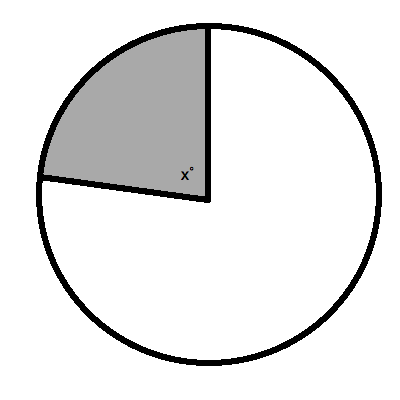The sector pictured above is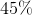of the circle. What is the angle measurefor the sector?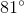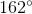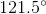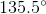Explanation:

A question like this is very easy. You merely need to find out what isof the total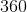degrees in a circle. This is: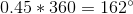. That is it!

### Example Question #2 : Sectors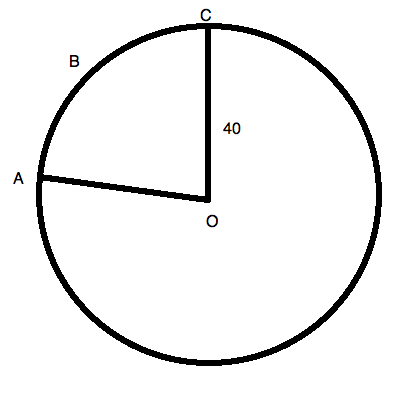The area of sector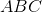is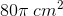. This figure is not drawn to scale.

What is the measure of the angle of the sector?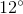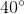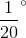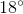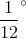Explanation:

You know that the area of a circle is computed by the equation:For our data, this is: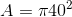or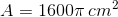Now, the sector is a percentage of the circle. For the areas, this can be represented as the fraction: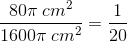The total degree measure of a circle is, of course,degrees.  This means that the sector contains: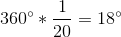.

### Example Question #3 : Sectors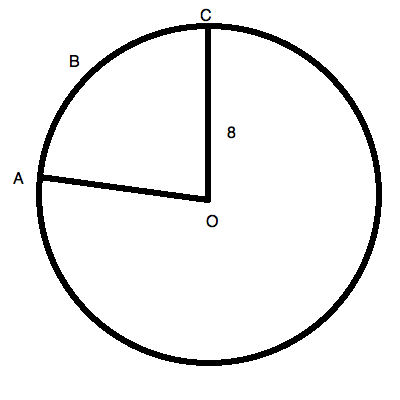The arc length of sector above is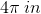. This figure is not drawn to scale.

What is the angle measure of sector?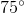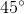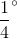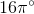Explanation:

You know that the circumference of a circle is computed by the equation:For our data, this is: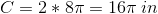Now, the sector is a percentage of the circle. For the lengths of the circumference and the arc length, this can be represented as the fraction: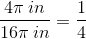The total degree measure of a circle is, of course,degrees. This means that the sector contains: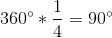.

### Example Question #1 : Sectors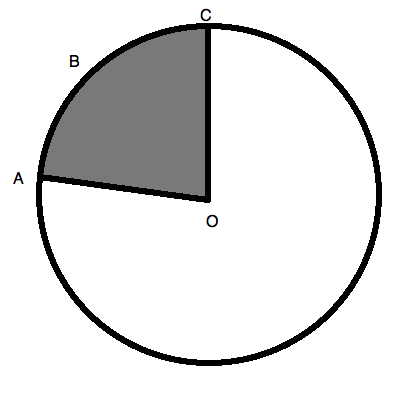Sectoris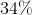of the total circle. This figure is not drawn to scale.

What is the angle of this sector?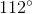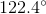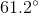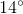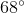Explanation:

Do not overthink this question! All you need to remember is that a given circle containsdegrees. This means that the sector is merely a percentage of. For our question, this percentage is, which is the same as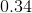. So, to calculate, you merely need to multiply: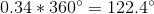This is the degree measure of the sector.

### Example Question #5 : Sectors

A bike wheel hasevenly spaced spokes spreading from its center to its tire. What must the angle be for the spokes in order to guarantee this even spacing? Round to the nearest hundredth.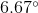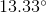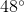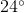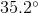Remember that the total degree measure of a circle is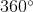. This means that if you haveparts into which you have divided your circle, each spoke must be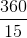orapart.